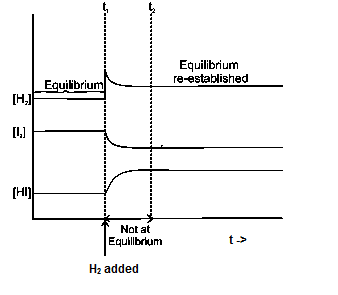# Le Chatelier's Principle

## Le Chatelier's Principle:-

• In a system under equilibrium if there is change in pressure, temperature or concentration then the equilibrium shifts in such a manner as to reduce or counteract the reflect of change.
• We will examine the impact of temperature , pressure , change in concentration , addition of catalyst in the chemical reaction based on that

### Effect of temperature

Equilibrium constant value depends on the temperature. Also the temperature dependence of the equilibrium constant depends on the sign of $\Delta H$ for the reaction
a. The equilibrium constant for an exothermic reaction (negative $\Delta H$ ) decreases as the temperature increases.
b. The equilibrium constant for an endothermic reaction (positive $\Delta H$ ) increases as the temperature increases.
So , On increasing temperature equilibrium shifts towards endothermic direction.
Example
$N_2 (g) + 3H_2 (g) \rightleftharpoons 2NH_3 (g)$
$\Delta H = -92.38 KJ/mol$
So as per Le Chatelier's Principle, on increasing the temperature , the equibrium will shift towards left and less ammonia will be formed

### Effect of pressure

On increasing pressure equilibrium shifts where no.of moles are less.
$CO(g) + 3H_2 (g) \rightleftharpoons CH_4 (g) + H_2O(g)$
Here reactants =4 moles
Products =2 moles
So ,on increasing the pressure, the equilibrium will shifts towards less mole side. So it will move in the forward direction

### Effect of change in concentration

On change in concentration equilibrium shifts to compensate the change. When the concentration of any of the reactants or products in a reaction at equilibrium is changed, the composition of the equilibrium mixture changes so as to minimize the effect of concentration changes
If we add more reactants or products , the equilibrium will shifts in such a manner so that add products or reactants are consumed
If we remove reactants or products , the equilibrium will shifts in such a manner so that remove products or reactants are replenished
Example
$H_2 (g) + I_2 (g) \rightleftharpoons 2HI(g)$
If H2 is added to the reaction mixture at equilibrium, then the equilibrium of the reaction is disturbed. In order to restore it, the reaction proceeds in a direction wherein H2 is consumed, i.e., more of H2 and I2 react to form HI and finally the equilibrium shifts in right (forward) direction## Effect of catalyst

Catalyst increases both forward & backward reaction at the same rate.
Therefore , No. effect or equilibrium